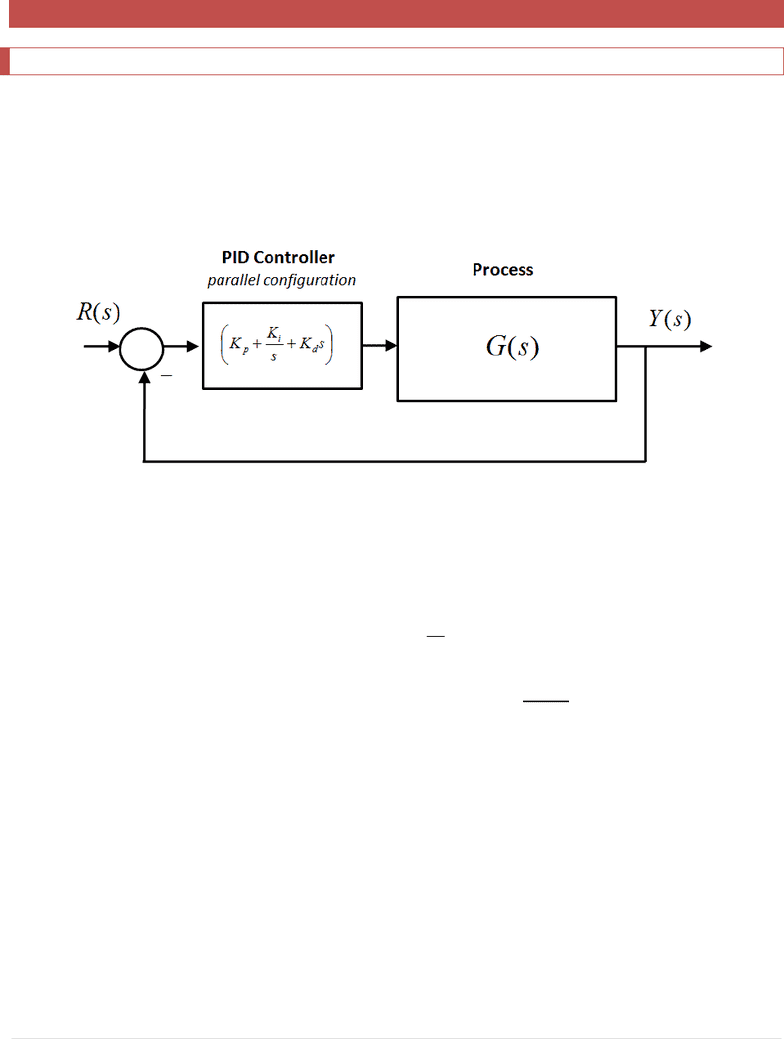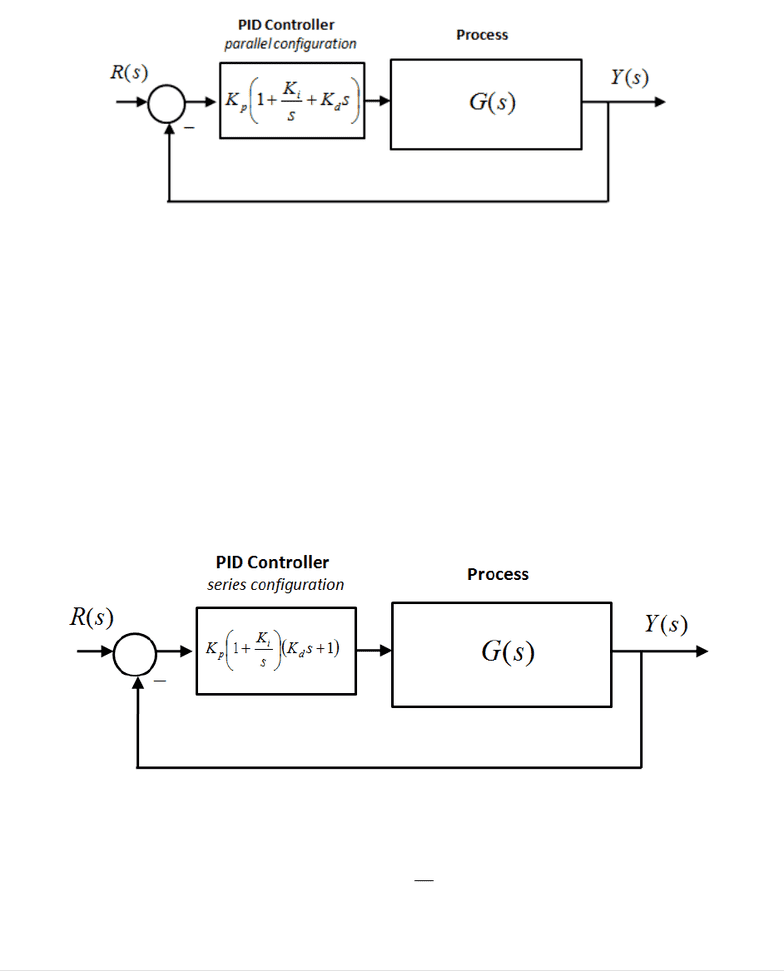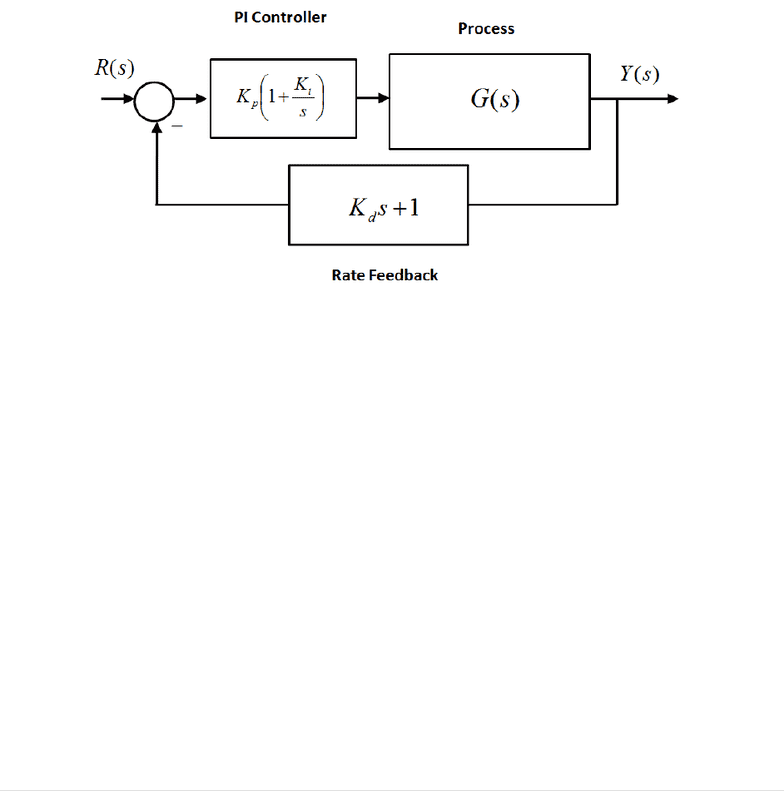# ELE 639 Lecture Notes - Lecture 9: Frequency Response, Loop Gain, Pid Controller

11 views23 pages
School
Course
ProfessorELE639 Course Notes Winter 2018
159 | P a g e
9 Three Mode Controller - PID Control
9.1 Introduction
One of the most versatile controller configurations for simple single feedback loop control is a PID Controller. We
already became familiar with the various forms of this controller both in the Lab as well as in two previous
Chapters, where we utilized the "Top-down" design approach to finding the controller gain values, based on the
understanding of the second order model and the effects of additional poles and zeros on the system response. In
this Chapter we will further discuss the properties of the three-mode controller (PID), including some of its
practical aspects.
Figure 9-1: Basic Unit Feedback Closed Loop System under PID Control (Parallel Structure)
The three-mode-controller name (PID) stands for Proportional + Integral + Derivative. Its parallel configuration is
realized as follows:
 
 

Equation 9-1
where e(t) is error, and u(t) is the Controller output, actuating the Process. Equation 9-1 represents the so-called
Parallel PID Structure, where the three modes of controller operation are added - the controller output is a sum of
the three control channels: P, I and D. The parallel structure is very easily implemented digitally. This form is also
very convenient for intuitive, step-by-step controller tuning where each mode is added independently. For more
on PID tuning, see appendices in Lab 2. The tuning approach, unlike the more analytical "top-down" design
approach, does not guarantee the "best possible" combination of parameters, but it provides a good starting point
for an analytical design where Root Locus Analysis or Frequency Response Analysis (more on Frequency Response
to come in Chapter 12) are considered.
Unlock document

This preview shows pages 1-3 of the document.
Unlock all 23 pages and 3 million more documents.ELE639 Course Notes Winter 2018
160 | P a g e
A variation of the Parallel Structure is also used, shown Figure 9-2, with the Proportional Controller Gain as a
multiplier - it is more useful when Root Locus Analysis is used (more on Root Locus to come in Chapter 10), as we
can vary the Proportional Gain - this is not possible for the classical Parallel Structure of Equation 9-1.
Figure 9-2: Basic Unit Feedback Closed Loop System under PID Control
However, the problem with the Parallel PID Structure is that the zeros of the closed loop transfer function, shown
in Equation 9-1, interact, since they are solutions of a quadratic term - when one zero location is changed (i.e. the
value of one of the time constants is changed), the other zero moves as well.
Therefore, for a more analytical approach (i.e. if we want to use the Root Locus, or Frequency Response plots to
design the PID Controller) this form is not so convenient: the two zeros interact, shifting at the same time. An
alternative configuration of the PID Controller can be implemented, called the Series PID Controller Structure,
shown in Figure 9-3 and in Equation 9-2. The zeros of the closed loop transfer function are now independent and
can be moved on the Root Locus or on the Frequency Response plot one at a time.
Figure 9-3 Series PID Controller Structure
 
Equation 9-2
Unlock document

This preview shows pages 1-3 of the document.
Unlock all 23 pages and 3 million more documents.ELE639 Course Notes Winter 2018
161 | P a g e
Finally, when the Series PID structure is divided into two terms, one in the forward path and one in the feedback
path, we have the PI + Rate Feedback structure as shown in Figure 9-4.
Both the Parallel and the Series PID Structures have two zeros, and therefore contribute these two zeros to the
closed loop transfer function. In case of the PI + Rate Feedback, only one zero is contributed to the closed loop
transfer function. The PI + Rate Feedback Structure can be used if the Derivative effect has to be reduced because
either we have a large additional overshoot in the step response because the system zeros are in the dominant
region, or a wind-up effect is taking place, or when we are dealing with a noisy environment, since the Bandwidth
of the system with only one zero is smaller than the Bandwidth of the system with two zeros and thus the
configuration with the Rate Feedback provides more attenuation for noise, which is usually a higher frequency
phenomenon.
Figure 9-4 PI + Rate Feedback Controller Structure
In general, when dealing with a system under PID Control, we take the following steps:
Obtain a closed loop system that is stable
Exert a reasonable level of control signal to the process - the Proportional Controller does most of the
work. Proportional Mode is the "work-horse", or the "muscle", of the PID Controller. Proportional Mode is
used to provide stable, fast and accurate response.
Integral and Derivative Gains should be used sparingly, to make subtle adjustments in the system
response:
o Integral Mode increases the System Type and therefore reduces the steady state error - PI
(Proportional + Integral) Control is used to improve steady state tracking.
o Derivative Mode is used to increase damping - PD (Proportional + Derivative) Control is used to
increase damping and therefore to decrease oscillations and also to speed up the system
response.
Fine-tune as required during implementation by adding the Integral and Derivative action.
An important observation about the PID Control - the Integral and Derivative action should be used sparingly! A
nice analogy for the Controller settings is to think about the Proportional Control as a main meal, while the Integral
Unlock document

This preview shows pages 1-3 of the document.
Unlock all 23 pages and 3 million more documents.

## Document Summary

One of the most versatile controller configurations for simple single feedback loop control is a pid controller. We already became familiar with the various forms of this controller both in the lab as well as in two previous. Chapters, where we utilized the top-down design approach to finding the controller gain values, based on the understanding of the second order model and the effects of additional poles and zeros on the system response. In this chapter we will further discuss the properties of the three-mode controller (pid), including some of its practical aspects. Figure 9-1: basic unit feedback closed loop system under pid control (parallel structure) The three-mode-controller name (pid) stands for proportional + integral + derivative. Its parallel configuration is realized as follows: (cid:3005)(cid:4666)(cid:1871)(cid:4667)=(cid:3043)+(cid:1871)+(cid:3031)(cid:1871) (cid:1873)(cid:4666)(cid:1872)(cid:4667)=(cid:3043) (cid:1857)(cid:4666)(cid:1872)(cid:4667)+ (cid:1857)(cid:4666)(cid:1872)(cid:4667)(cid:1856)(cid:1872)+(cid:3031)(cid:1856)(cid:1857)(cid:4666)(cid:1872)(cid:4667)(cid:1856)(cid:1872) Equation 9-1 where e(t) is error, and u(t) is the controller output, actuating the process.

## Get access

\$8 USD/m\$10 USD/m
Billed \$96 USD annuallyHomework Help
Study Guides
Textbook Solutions
Class Notes
Textbook Notes
Booster Class